Courses

# 28 Year NEET Questions: States Of Matter- 1

## 17 Questions MCQ Test Chemistry 28 Years Past year papers for NEET/AIPMT Class 11 | 28 Year NEET Questions: States Of Matter- 1

Description
This mock test of 28 Year NEET Questions: States Of Matter- 1 for NEET helps you for every NEET entrance exam. This contains 17 Multiple Choice Questions for NEET 28 Year NEET Questions: States Of Matter- 1 (mcq) to study with solutions a complete question bank. The solved questions answers in this 28 Year NEET Questions: States Of Matter- 1 quiz give you a good mix of easy questions and tough questions. NEET students definitely take this 28 Year NEET Questions: States Of Matter- 1 exercise for a better result in the exam. You can find other 28 Year NEET Questions: States Of Matter- 1 extra questions, long questions & short questions for NEET on EduRev as well by searching above.
QUESTION: 1

### Which of the following expressions correctly represents the relationship between the average molar kinetic energy,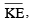of  CO and N2 molecules at the same temperature ?

Solution: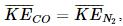because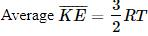It does not depend upon the nature of the gas.

QUESTION: 2

### Van der Waal's real gas, act as an ideal gas, at which conditions? 

Solution:

At higher temperature and low pressure real gas acts as an ideal gas

QUESTION: 3

### The surface tension of which of the following liquid is maximum? 

Solution:

Due to intermolecular H-bonding the surface tension of H2O is more than other liquid. One H2O molecule is joined with 4 another H2O molecule through H–bond.
Hydrogen bonding is in order H2O > C2H5OH > CH3OH.

QUESTION: 4

If a gas expan ds at constan t temperature, it indicates that : 

Solution:

At an y constan t temper ature the K.E. of gaseous molecules remains same. (K.E. ∝ T).
Thus option (c) is correct answer.

QUESTION: 5

The pressure exerted by 6.0g of methane gas in a 0.03 m3 vessel at 129°C is (Atomic masses : C = 12.01, H = 1.01 and R = 8.314 JK–1 mol –1)

Solution: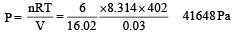QUESTION: 6

Three moles of an idea l gas expanded spontaneously into vacuum. The work done will be :

Solution:

Ideal gas during spontaneous expansion into vacuum does not do any external work.

QUESTION: 7

By what factor does the average velocity of a gaseous molecule increase when the temperature (in Kelvin) is doubled ? 

Solution:

Average velocity =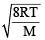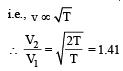QUESTION: 8

Two gases A and B having the same volume diffuse through a porous partition in 20 and 10 seconds respectively. The molecular mass of A is 49 u. Molecular mass of B will be : 

Solution: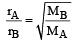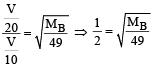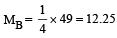QUESTION: 9

A gaseous mixture was prepared by taking equal mole of CO and N2. If the total pressure of the mixture was found 1 atmosphere, the partial pressure of the nitrogen (N2) in the mixture is :

Solution:

Given nCO = nN2 PCO + PN2 = 1 atm

Partial pressure of a gas = mole fraction of gas × total pressure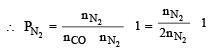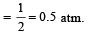QUESTION: 10

Which of the following is correct option for free expansion of an ideal gas under adiabatic condition ? 

Solution:

For free expansion of an ideal gas under adiabatic condition q = 0, ΔT = 0 , w = 0

QUESTION: 11

A bubble of air is underwater at temperature 15°C and the pressure 1.5 bar. If the bubble rises to the surface where the temperature is 25°C and the pressure is 1.0 bar, what will happen to the volume of the bubble ? [2011M]

Solution:

Given 1 = 1.5 bar T1 = 273 + 15 = 288 K V1 = V
P2 = 1.0 bar T1 = 273 + 25 = 298K V2 = ?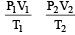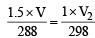V2 = 1.55 V i.e., volume of bubble will be almost 1.6 time to initial volume of bubble.

QUESTION: 12

50 mL of each gas A and of gas B takes 150 and 200 seconds respectively for effusing through a pin hole under the similar condition. If molecular mass of gas B is 36, the molecular mass of gas A will be : 

Solution: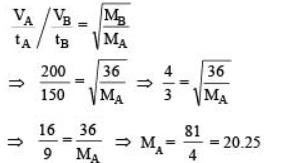QUESTION: 13

certain gas takes three times as long to effuse out as helium. Its molecular mass will be

Solution: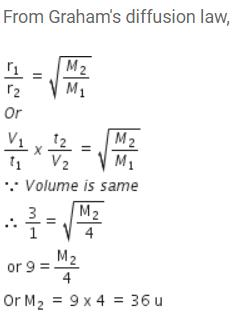QUESTION: 14

For real gases van der Waals equation is written as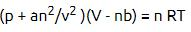where ‘a’ and ‘b’ are van der Waals constants.
Two sets of gases are :

(I) O2, CO2, H2 and He

(II) CH4, O2 and H2

The gases given in set-I in increasing order of ‘b’ and gases given in set-II in decreasing order of ‘a’, are arranged below. Select the correct order from the following :

Solution:

Van der Waal gas constant 'a' represent intermolecular force of attraction of gaseous molecules and Van der Waal gas constant 'b' represent effective size of molecules. Therefore order should be (I) H2 < He < O2 < CO2

(II) CH4 > O2 > H2

QUESTION: 15

Maximum deviation from ideal gas is expected from : [NEET 2013]

Solution:

Higher the critical temperature more easily will be the gas liquify.  Now since most easily liquifiable gas show larger deviation, NH3 will show maximum deviation from ideal behaviour.

QUESTION: 16

Dipole-induced dipole interactions are present in which of the following pairs : [NEET 2013]

Solution:

This type of attractive force operates between the polar molecules having permanent dipole and the molecules lacking permanent dipole.
HCl is polar (μ ≠ 0) and He is non polar (μ = 0), thus gives dipole-induced dipole interaction.

QUESTION: 17

What is the density of N2 gas at 227°C and 5.00 atm pressure? (R = 0.0821 atm K–1 mol–1) [NEET Kar. 2013]

Solution:

Density =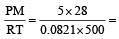3.41g/ml